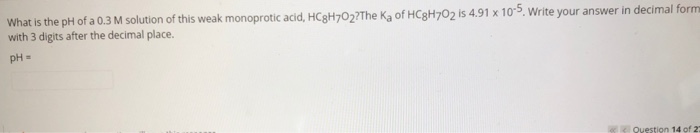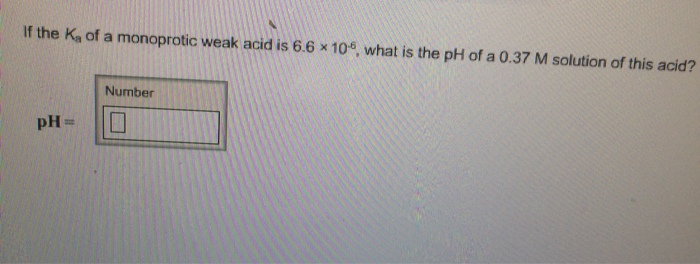# Find the pH of a 0.100 M solution of a weak monoprotic acid having Ka= 1.2×10−3....

Find the pH of a 0.100 M solution of a weak monoprotic acid having Ka= 1.2×10−3.

Let the acid be HA

HA----> H+ +A-

Initial   HA = 0.1    H+ = [A-]= 0

change [HA] =-x   [H+] =[A-] =x

At Equilibrium   HA= 1-x       [H+] =[A-] =x

Ka= x2/(1-x)= 1.2*10-3

this equation gives when solved usong solved gives x =0.0341

[H+] =0.0341

pH= -log(0.0341)=1.467

#### Earn Coin

Coins can be redeemed for fabulous gifts.

Similar Homework Help Questions
• ### Find the pH of a 0.130 M solution of a weak monoprotic acid having Ka= 1.1×10−3....

Find the pH of a 0.130 M solution of a weak monoprotic acid having Ka= 1.1×10−3. Express your answer to two decimal places.

• ### A) Find the pH of a 0.120 M solution of a weak monoprotic acid having Ka=...

A) Find the pH of a 0.120 M solution of a weak monoprotic acid having Ka= 1.5×10−5. B) Find the percent dissociation of this solution. C) Find the pH of a 0.120 M solution of a weak monoprotic acid having Ka= 2.0×10−3. D) Find the percent dissociation of this solution. E) Find the pH of a 0.120 M solution of a weak monoprotic acid having Ka= 0.14. F ) Find the percent dissociation of this solution.

• ### Find the pH and percent ionization of a 0.100 M solution of a weak monoprotic acid...

Find the pH and percent ionization of a 0.100 M solution of a weak monoprotic acid having the given Ka values. (a) Ka = 1.9 10-5 (b) Ka = 1.9 10-3 (c) Ka = 1.9 10-1

• ### Find the pH of a 0.140 M solution of a weak monoprotic acid having Ka values.

1. Find the pH of a 0.140 M solution of a weak monoprotic acid having Ka =1.7×10^-3.and Find the percent dissociation of this solution.I got pH=1.84 for the first one and it was correct.I got 11% of the second answer but it was incorrect...2. Find the pH of a 0.140 M solution of a weak monoprotic acid having Ka = 0.11.and Find the percent dissociation of this solution.I got 0.91 for the first one and it was incorrect....I don't know...

• ### What is the pH of a 0.3 M solution of this weak monoprotic acid, HC3H702?The Ka...What is the pH of a 0.3 M solution of this weak monoprotic acid, HC3H702?The Ka of HC3H702 is 4.91 x 10-5. Write your answer in decimal form with 3 digits after the decimal place. pH- Question 14 of 2

• ### What is the pH of a solution of 40.0 mL of 0.100 M acetic acid (Ka...What is the pH of a solution of 40.0 mL of 0.100 M acetic acid (Ka = 1.8 x 10-5) after 50.0 mL of 0.100 M NaOH has been added? Calculate the concentration of dissolved Ba2+ ions when BaSO4 is added to water at 25°C. Кsp? = 1.10 x 10-10 A particular saturated solution of silver chromate (Ag2CrO4), has [Ag+] = 5.0 x 10 Mand (CrO4) = 4.4 x 10M. What is value Ksp for silver chromate? As a result...

• ### If the Ka of a monoprotic weak acid is 6.4 × 10-6, what is the pH...

If the Ka of a monoprotic weak acid is 6.4 × 10-6, what is the pH of a 0.39 M solution of this acid?

• ### If the Ka of a monoprotic weak acid is 6.6*10^6, what is the pH of a...If the Ka of a monoprotic weak acid is 6.6*10^6, what is the pH of a 0.37 M solution of this acid?

• ### If the Ka of a monoprotic weak acid is 3.0 × 10-6, what is the pH...

If the Ka of a monoprotic weak acid is 3.0 × 10-6, what is the pH of a 0.45 M solution of this acid?

• ### If the Ka of a monoprotic weak acid is 4.7 × 10-6, what is the pH...

If the Ka of a monoprotic weak acid is 4.7 × 10-6, what is the pH of a 0.46 M solution of this acid?